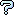All about flooble | fun stuff | Get a free chatterbox | Free JavaScript | Avatarsperplexus dot infoRARE SURE QUORA (Posted on 2011-10-02)How much is OPEN*AT if the partial products are ERROR & REAPS ?

 See The Solution Submitted by Ady TZIDON Rating: 5.0000 (1 votes)Comments: ( Back to comment list | You must be logged in to post comments.)Is there a bug in my program?| Comment 2 of 7 |My program fails to find any results. It's designed to look at all valid values of O, P, E, N, A and T, and deduce fitting values for R and S, but fails to find any.

Either there's a bug in my program or this is not a traditional alphametic (cryptarithm).

DEFDBL A-Z
FOR o = 1 TO 9
IF used(o) = 0 THEN
used(o) = 1
FOR a = 1 TO 9
IF used(a) = 0 THEN
used(a) = 1
FOR p = 0 TO 9
IF used(p) = 0 THEN
used(p) = 1
FOR e = 0 TO 9
IF used(e) = 0 THEN
used(e) = 1
FOR n = 0 TO 9
IF used(n) = 0 THEN
used(n) = 1
FOR t = 0 TO 9
IF used(t) = 0 THEN
used(t) = 1
openval = o * 1000 + p * 100 + e * 10 + n
pp1 = openval * t: pp2 = openval * a
tst1 = pp1 - e * 10000 - o * 10
r = tst1 / 1101
IF r = INT(r) AND r < 10 AND r > 0 THEN
IF used(r) = 0 THEN
used(r) = 1
tst2 = pp2 - r * 10000 - e * 1000 - a * 100 - p * 10
IF tst2 > 0 AND tst2 < 10 THEN
IF used(tst2) = 0 THEN
PRINT openval; "*"; a * 10 + t; "="; openval * (10 * a + t)
END IF
END IF
used(r) = 0
END IF
END IF
SWAP pp1, pp2
tst1 = pp1 - e * 10000 - o * 10
r = tst1 / 1101
IF r = INT(r) AND r < 10 AND r > 0 THEN
IF used(r) = 0 THEN
used(r) = 1
tst2 = pp2 - r * 10000 - e * 1000 - a * 100 - p * 10
IF tst2 > 0 AND tst2 < 10 THEN
IF used(tst2) = 0 THEN
PRINT openval; "*"; a * 10 + t; "="; openval * (10 * a + t)
END IF
END IF
used(r) = 0
END IF
END IF
used(t) = 0
END IF
NEXT
used(n) = 0
END IF
NEXT
used(e) = 0
END IF
NEXT
used(p) = 0
END IF
NEXT
used(a) = 0
END IF
NEXT
used(o) = 0
END IF
NEXT

 Posted by Charlie on 2011-10-03 10:38:56Please log in:

 Search: Search body:
Forums (0)